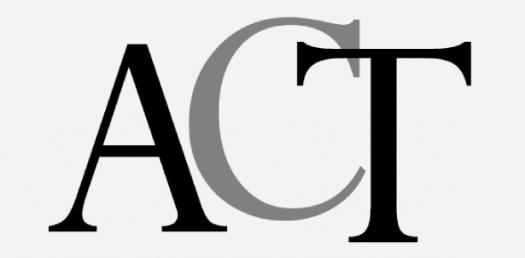# Math Trivia Quiz: Act 5-in-5

Approved & Edited by ProProfs Editorial Team
The editorial team at ProProfs Quizzes consists of a select group of subject experts, trivia writers, and quiz masters who have authored over 10,000 quizzes taken by more than 100 million users. This team includes our in-house seasoned quiz moderators and subject matter experts. Our editorial experts, spread across the world, are rigorously trained using our comprehensive guidelines to ensure that you receive the highest quality quizzes.
| By Melacat
M
Melacat
Community Contributor
Quizzes Created: 4 | Total Attempts: 2,912
Questions: 5 | Attempts: 821Settings.

• 1.

### The expression a[b + (c – d)] is equivalent to:

• A.

A. ab + ac – ad

• B.

B. ab + abc – ad

• C.

C. ab + ac – d

• D.

D. ab + c + d

• E.

E. ab + c – d

A. A. ab + ac – ad
Explanation
The given expression a[b + (c - d)] can be simplified by applying the distributive property. Distributing the a to both terms inside the brackets, we get ab + (a * (c - d)). Simplifying further, we have ab + ac - ad, which matches option A. Therefore, the correct answer is A. ab + ac - ad.

Rate this question:

• 2.
• A.

• B.

• C.

• D.

• E.

C.
• 3.

### So far, a student has earned the following scores on four 100-point tests this grading period:  65, 73, 81, and 82.  What score must the student earn on the fifth and last 100-point test of the grading period to earn an average test grade of 80 for the 5 tests?

• A.

75

• B.

76

• C.

84

• D.

99

• E.

The student cannot earn an average score of 80.

D. 99
Explanation
To find the score the student must earn on the fifth test to have an average of 80, we can use the formula: (sum of scores + score on fifth test) / 5 = 80. Rearranging the formula, we get: sum of scores + score on fifth test = 400. The sum of the scores on the first four tests is 65 + 73 + 81 + 82 = 301. Therefore, the score the student must earn on the fifth test is 400 - 301 = 99.

Rate this question:

• 4.

### A circle in the standard (x, y) coordinate plane is tangent to the x-axis at 5 and tangent to the y-axis at 5.  Which of the following is an equation of the circle?

• A.

• B.

• C.

• D.

• E.

D.
Explanation
The equation of a circle can be written in the form (x - h)^2 + (y - k)^2 = r^2, where (h, k) is the center of the circle and r is the radius. Since the circle is tangent to the x-axis at 5, the y-coordinate of the center is 5. Similarly, since the circle is tangent to the y-axis at 5, the x-coordinate of the center is 5. Therefore, the center of the circle is (5, 5). The radius of the circle can be found by measuring the distance from the center to either tangent point, which is 5 units. Thus, the equation of the circle is (x - 5)^2 + (y - 5)^2 = 25.

Rate this question:

• 5.

### What 2 numbers should be placed in the blanks below so that the difference between consecutive numbers is the same

• A.

A. 23, 29

• B.

B. 24, 34

• C.

C. 25, 33

• D.

D. 26, 35

• E.

E. 27, 31

C. C. 25, 33
Explanation
The numbers 25 and 33 should be placed in the blanks because the difference between them is 8, which is the same as the difference between the other pairs of consecutive numbers.

Rate this question:

Related TopicsBack to top